# Bitcoin Historical Volatility — Why the Calculation Method Matters

### Bitcoin Historical Volatility — Why the Calculation Method Matters

By Sacha Ghebali and Olivier Mammet

### Introduction

All Bitcoin traders and holders are well attuned to frequent, and at times violent, price swings. Let’s say a new buyer experiences a 10% overnight price jump — good news! But the next day, the price falls 16%. This buyer may now be frantically checking their wallet to decide if they should cut losses and revisit their investment strategy. But what if the price had only moved in increments of 0.1%? Less attention would likely be devoted to watching the price. This is precisely what volatility measures: How much variation there is in consecutive price changes over time.

In this article, a few methods for calculating historical volatility are reviewed and an important note of caution is drawn on the interpretation of sudden drops in volatility that have been shown on various crypto research pieces in the recent past.

### Key takeaways:

• Historical volatility is a backward-looking measure on which relatively old events can have large effects. Therefore, it is important to carefully understand the calculation methodology to make informed decisions.
• It is possible to have a more nimble measure of volatility by putting more weight on recent returns.
• An exponentially moving average with a decay factor of 0.8 was shown to be reactive to the recent rapid market activity whilst retaining relatively low noise.
• Excluding weekends and holidays in the volatility calculation did not have a significant impact using the proposed methodology over the timeframe studied (Jan 1st — May 1st).

### Simple methodology

The simplest form to calculate the historical volatility, also sometimes called realized volatility, is to estimate the standard deviation of the log price returns, with log returns being defined as:

Where r is the log return, P is the price, and t is the time instant. For daily returns, there are 24h between t and t-1. Here, the price is calculated from an average of the midnight UTC price, weighted by the daily volume across major exchanges (cf. PDF version of our research factsheet for further details on calculations, and for the list of selected exchanges).

An example of natural returns (slightly different from log returns but very close in value when returns are small — for example, a natural return of 10% corresponds to a log return of about 9.5%) calculated from this aggregated price for Bitcoin can be found on our weekly Research Factsheet as shown below in Figure 1.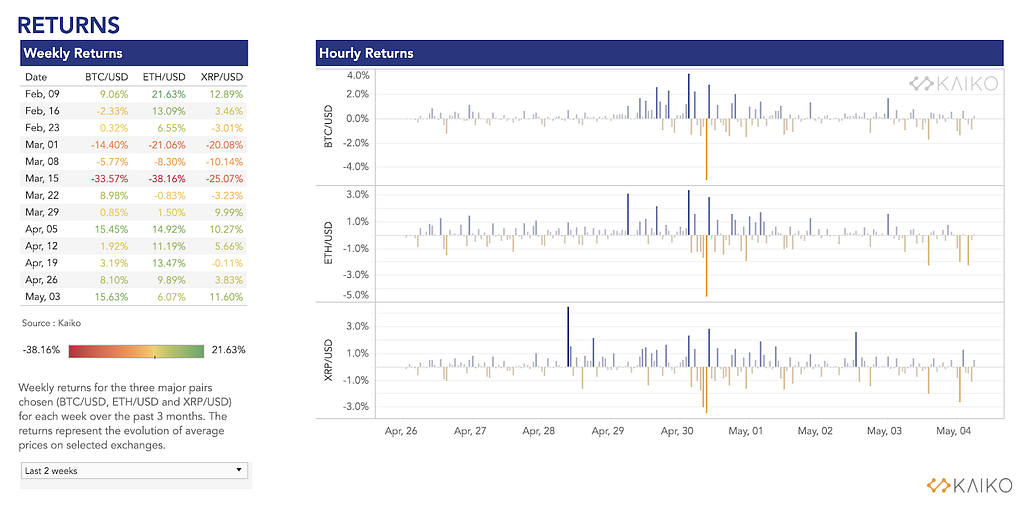Figure 1: Weekly and hourly natural returns for BTC/USD, ETH/USD and XRP/USD (source: Research Factsheet).

Next, the realized volatility is calculated by taking the rolling standard deviation over a fixed time window (multiplied by a coefficient to normalize the variations to annual volatility, more on this later). It is common to use a window of the order of 20 days to a month. Too short of a window and there will be too much noise resulting from the lack of samples, too long of a window and the volatility estimate will fail to adapt to recent market movements.

As shown in Figure 2, the simple realized volatility on a 20-day window displays two sudden jumps: one on March 12th (the famous ‘Black Thursday’), and another twenty days later when the volatility suddenly drops. Coincidence with the 20-day window?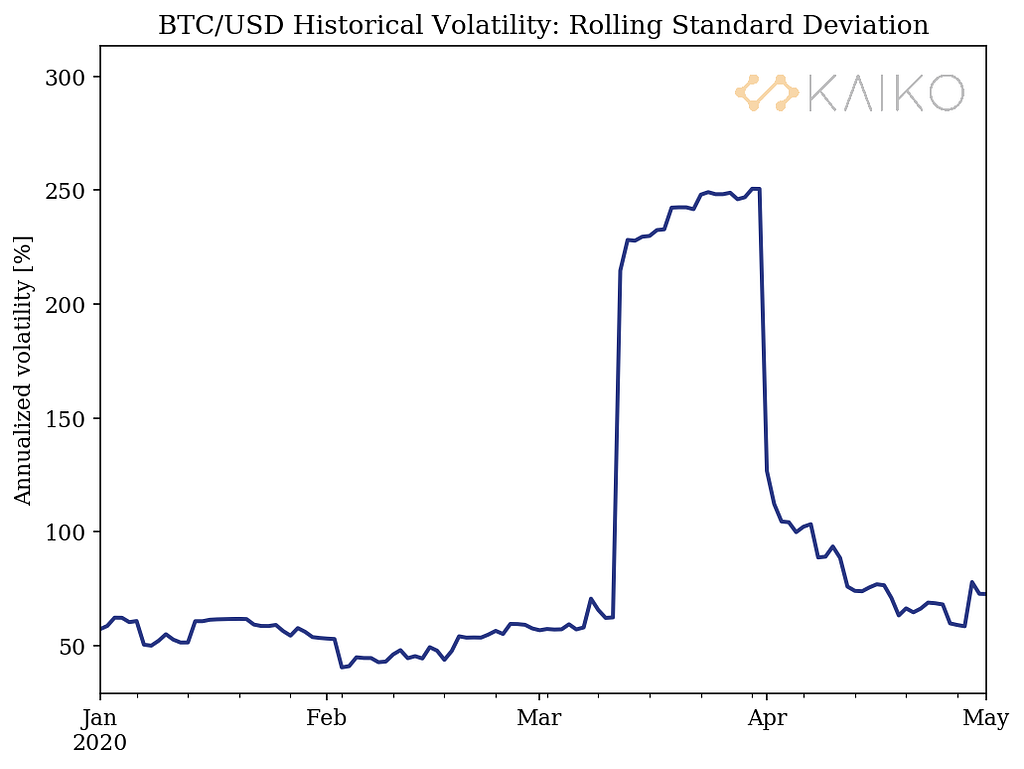Figure 2: BTC/USD historical volatility computed from a 20-day rolling standard deviation.

In order to understand the second jump, it is necessary to look at the returns over time, as shown in Figure 3. On March 12th the volatility suddenly increased because of the large return of about -50%; twenty days later, this value of -50% goes out of the window and is no longer included in the calculation of the standard deviation, causing a drop in volatility accordingly.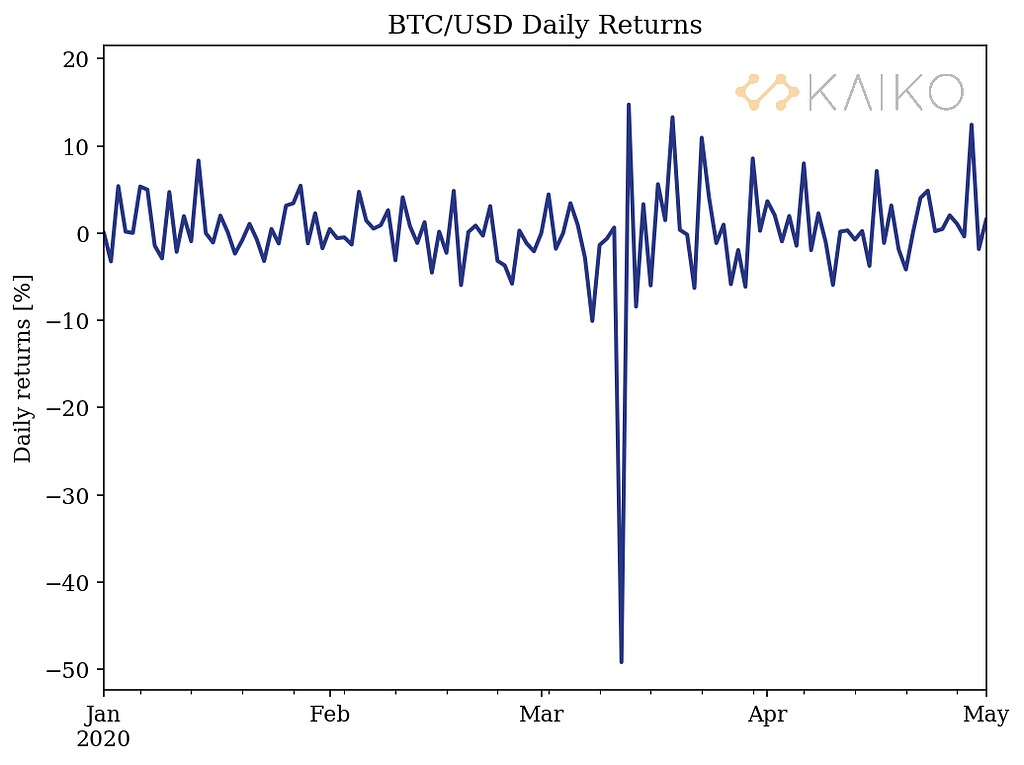Figure 3: Daily log returns for BTC/USD, calculated from an end-of-day volume-averaged price across major exchanges at midnight UTC.

This simple example illustrates the importance of understanding how volatility is calculated in the interpretations that one draws from the numbers. The following section introduces an exponential weighting in order to reduce the impact of old events on the calculation of the historical volatility.

### Reducing the weight of old events

In order to smooth out the volatility curve, one common approach is to use what is called an exponentially weighted moving average (EWMA). The approach is quite simple: old returns will get a smaller weight than recent returns in the calculation. Even more powerful: this definition includes a relaxation parameter lambda which can be tuned to increase or reduce the weight placed on recent events relative to older values.

In practice, the EWMA estimate of the volatility (sigma) can be estimated recursively via the following formula:

Where lambda is the decay factor which can be controlled. A small value of lambda corresponds to strong decay and underweights old events more strongly. Conversely, a large value of lambda corresponds to weak decay, it improves the smoothness but slows the response time to market changes.

What should the value of this decay factor be? As mentioned, there is a tradeoff between having a value that is reactive to rapidly changing market conditions and having a precise smooth curve.

Multiple approaches can be used to set the value of the decay factor. One solution is to consider a maximum likelihood estimate, that is the value that maximizes the likelihood of the historical observations. An alternative used by RiskMetrics  attempts to minimize the forecast error and suggests the use of a value of 0.94.

In times of high market uncertainty, it may make sense to tune the value of the decay factor to a lower value in order to put more weight on recent events and consequently have a more adaptive measure of volatility. Figure 4 shows a comparison of the regular 20-day moving average (MA), an exponentially weighted moving average (EWMA) using the RiskMetrics decay factor, and finally an EWMA with a decay factor of 0.8 in order to increase the emphasis on recent events.

As shown in Figure 4, using a decay factor of 0.8 provides a measure of volatility that is closer to the rolling standard deviation than using the decay factor used by RiskMetrics. It also prevents the sharp, and arguably misleading, drop in volatility obtained in the case of the standard 20-day moving average.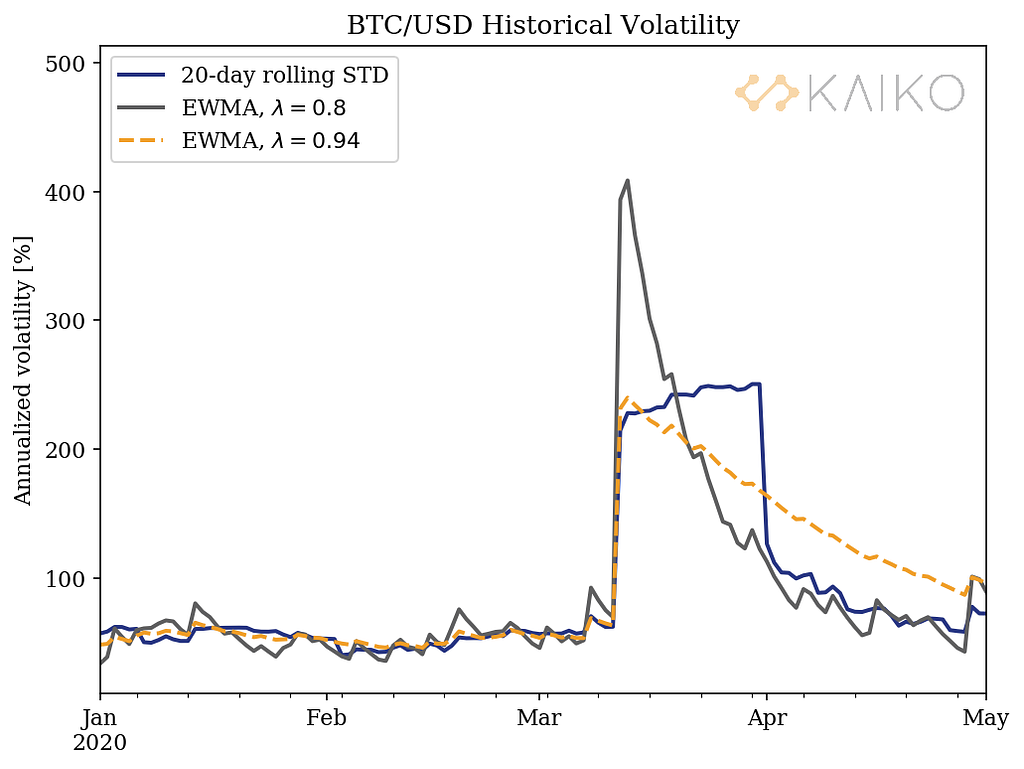Figure 4: Comparison of the BTC/USD historical volatility calculated from various methods.

### Number of open trading days

Finally, more detail on the annualization of the volatility is presented. First, why annualize? The main reason is that investors are used to analyzing annualized values. Annualized values also enable investors to compare volatility levels between sampling frequencies (e.g. daily, hourly, monthly, etc.). The longer the time interval, the larger the volatility, which increases with the square root of the time.

Thus, in order to annualize daily returns, one must multiply the daily volatility by the square root of the number of days in a year.

But here comes a question: traditional markets are not open seven days a week, rather they are approximately 252 trading days per year, so it is common practice to annualize daily volatility by simply multiplying by a factor of √252. Yet, crypto markets are active every single day of the year so it would require to be annualized by a factor √365.

The above observation might lead to differences when trying to compare the volatility of crypto assets to that of traditional markets. This effect was analyzed by comparing two calculation methodologies (using the EWMA with a decay factor of 0.8 introduced earlier):

1. Annualized volatility based on all daily returns and scaled by a factor √365.
2. Annualized volatility based only on open trading days and therefore scaled by a factor √252. Note that BTC/USD returns are calculated on 24h intervals and exclude non-trading days.

Figure 5 shows that both calculations give similar results which is comforting for comparing volatility levels between traditional and crypto markets.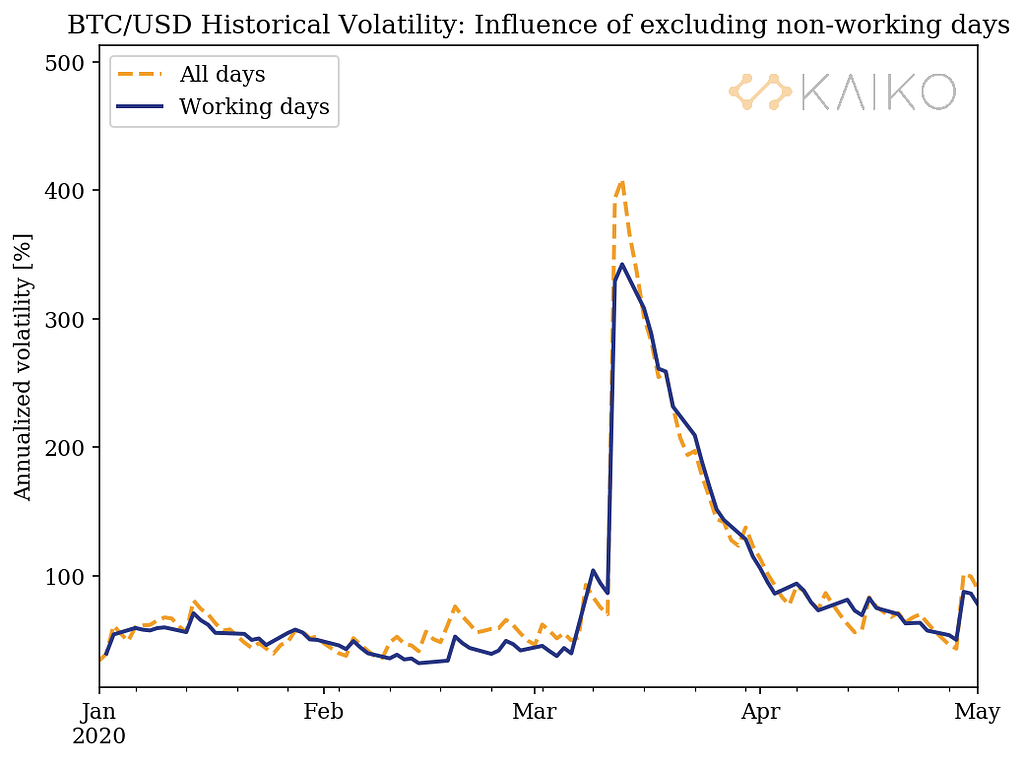Figure 5: Comparison of the BTC/USD historical volatility including/excluding non-working day for the EWMA with a decay factor of 0.8. The blue solid line only uses the returns observed on equity trading days and is annualized with a factor √252 ; the dashed orange line uses all daily returns and is annualized with a factor of √365.

As a matter of comparison, Figure 6 shows the exact same analysis but with the rolling standard deviation. The effect of excluding non-working days is larger than for the EWMA.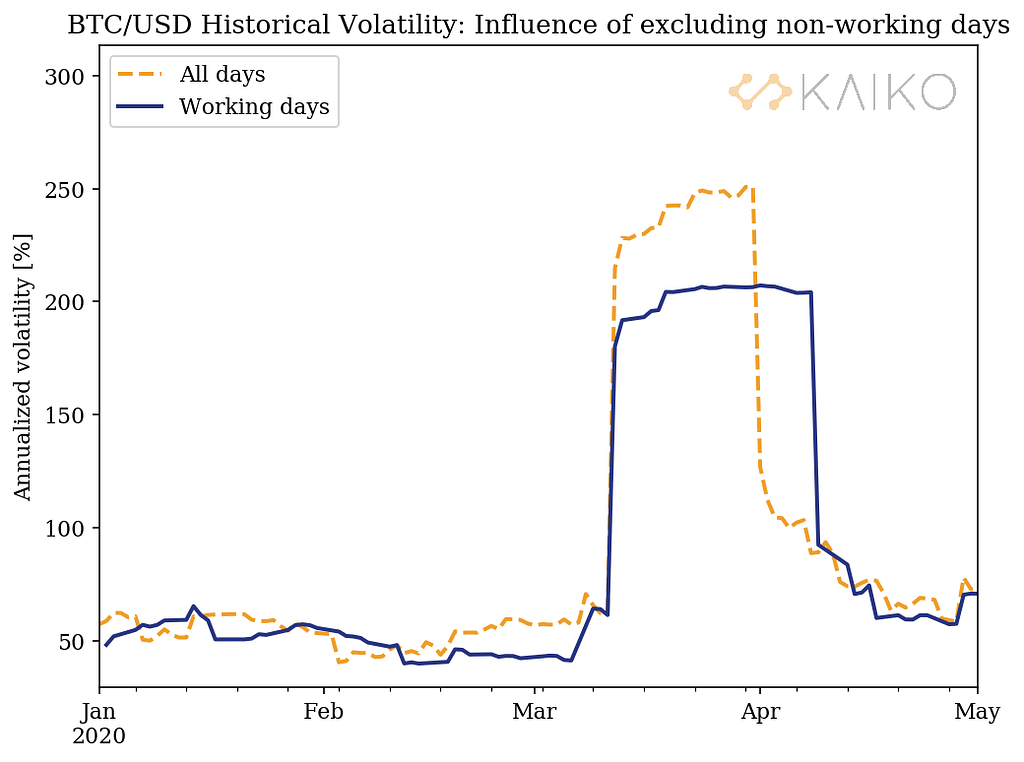Figure 6: Same as Figure 5 but using the 20-day rolling standard deviation method for the calculation of the historical volatility.

### Conclusions

Being able to understand the changes in volatility over time is important to inform investors’ trading and risk management decisions. The impact of the volatility calculation methodology was shown to be sizable in times of rapidly changing market conditions. The use of an exponentially weighted moving average with a decay factor of 0.8 was proposed to analyze the historical volatility following the crypto ‘Black Thursday’ of March 12th. However, it should be kept in mind that historical volatility provides a backward-looking view unlike other volatility measures like the CBOE’s volatility index (VIX).

In addition, the exclusion of weekends and bank holidays in the crypto returns was shown to yield reasonably little change in the calculation of the volatility with the EWMA with a decay factor of 0.8 over the time period studied.

### References

 RiskMetrics Group (2007), “The RiskMetrics 2006 methodology,” https://www.msci.com/documents/10199/d0905614-2771-46dc-b000-1a033146586aBitcoin Historical Volatility — Why the Calculation Method Matters was originally published in Kaiko Data on Medium, where people are continuing the conversation by highlighting and responding to this story.